# Floyd-Warshall Shortest Paths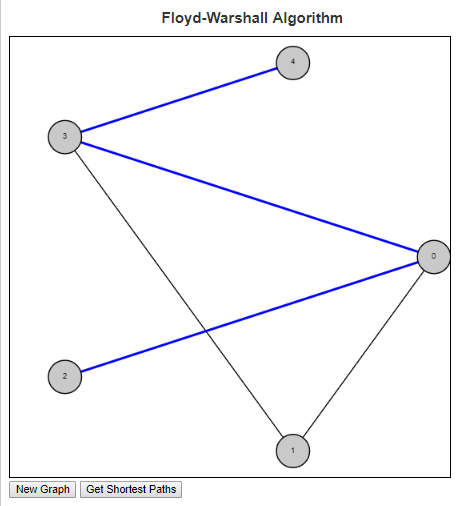The Floyd Warshall algorithm is an all pairs shortest paths algorithm. This can be contrasted with algorithms like Dijkstra’s which give the shortest paths from a single node to all other nodes in the graph.

Floyd Warshall’s algorithm works by considering first the edge set of the graph. This is the set of all paths of the graph through one edge. Node pairs that are connected to one another through an edge will have their shortest path set to the length of that edge, while all other node pairs will have their shortest path set to infinity. The program then runs through every triplet of nodes (i, j, k) and checks if the path from i to k and the path from k to j is shorter than the current path from i to j. If so, then the distance and the path is updated.

So lets consider an example on the graph in the image above. The edge set of this graph is E = {(0, 1), (0, 2), (0, 3), (1, 3), (3, 4)}. So our initial table is:

 0 1 2 3 4 0 inf (0, 1) (0, 2) (0, 3) inf 1 (0, 1) inf inf (1, 3) inf 2 (0, 2) inf inf inf inf 3 (0, 3) (1, 3) inf inf (3, 4) 4 inf inf inf (3, 4) inf

As we look to update the paths, we first look for routes that go through node 0:

Because node 0 connects to both node 1 and node 2, but node 1 does not connect to node 2, we have the following truth holding in the matrix above:
cost(0, 1) + cost(0, 2) < cost(1, 2), so we can update the shortest path from node 1 to node 2 to be (1, 0, 2).

Because node 0 connects to both node 2 and node 3, but node 2 does not connect to node 3, we have the following truth holding in the matrix above:
cost(0, 2) + cost(0, 3) < cost(2, 3), so we can update the shortest path from node 2 to node 3 to be (2, 0, 3).

Because node 3 connects to both node 0 and node 4, but node 0 does not connect to node 4, we have the following truth holding in the matrix above:
cost(0, 3) + cost(3, 4) < cost(0, 4), so we can update the shortest path from node 0 to node 4 to be (0, 3, 4).

Because node 3 connects to both node 1 and node 4, but node 1 does not connect to node 4, we have the following truth holding in the matrix above:
cost(1, 3) + cost(3, 4) < cost(1, 4), so we can update the shortest path from node 1 to node 4 to be (1, 3, 4).

Because node 3 connects to both node 2 and node 4, but node 2 does not connect to node 4, we have the following truth now holding:
cost(2, 3) + cost(3, 4) < cost(2, 4), so we can update the shortest path from node 2 to node 4 to be (2, 0, 3, 4).

The final table giving the list of shortest paths from every node to every other node is given below.

 0 1 2 3 4 0 inf (0, 1) (0, 2) (0, 3) (0, 3, 4) 1 (0, 1) inf (1, 0, 2) (1, 3) (1, 3, 4) 2 (0, 2) (1, 0, 2) inf (2, 0, 3) (2, 0, 3, 4) 3 (0, 3) (1, 3) (2, 0, 3) inf (3, 4) 4 (0, 3, 4) (1, 3, 4) (2, 0, 3, 4) (3, 4) inf

To see more examples and to help answer questions, check out the script in my examples section on the Floyd-Warshall algorithm

# Longest Common Subsequence

Suppose you and I each had an ordered list of items and we were interested in comparing how similar those lists are. One calculation we can perform on these two strings is the Longest Common Subsequence. A sequence X is an ordered list of elements <x1, …, xn>. A subsequence Z is another sequence where (1) Each element of Z is also an element of X and (2) The elements of Z occur in the same order (in Z) as they do in X.

Note that we do not say that the elements of Z need to be a continuous block of elements. If this were true we would be defining a substring. So as an example, suppose we have as an initial string,
X = C, O, M, P, U, T, E, R.
Then the following are all subsequences:
Z1 = C, M, U, T, R
Z2 = C, O, M, P
Z3 = U, T, E, R
Z4 = O, P, T, E

I will note that Z2 and Z3 are also substrings since they contain continuous sets of characters.

The length of a substring is simply the number of characters it contains. So X has length 8, Z1 has length 5, Z2, Z3 and Z4 have length 4.

Suppose now that we had a second string, Y = P, R, O, G, R, A, M, M, E, R and are interested in the longest common subsequence between the two. We can do that by observing that there is a bit of recursion going on with this question. What I mean by that is that asking the question of “What is the longest common subsequence between X and Y” is the same as asking “What is the longest common subsequence between X and Y once we have seen 8 characters of X and 10 characters of Y”

There are three possible ways to answer this question.

If X<sub>8</sub> is not equal to Y<sub>10</sub>, then the answer to this will be the same as the maximum of the pair X<sub>7</sub>, Y<sub>10</sub> and the pair X<sub>8</sub>, Y<sub>9</sub>.
If we reach a situation where we reach the beginning of either string, we are forced to answer 0 to that question.

Then the function has the following look:

LCS(Xi, Yj) =
 0, if i is 0 or j is 0 1 + LCS(Xi-1, Yj-1) if Xi equals Yj max(LCS(Xi-1, Yj), LCS(Xi, Yj-1))

Below is a table showing how we would solve the problem mentioned.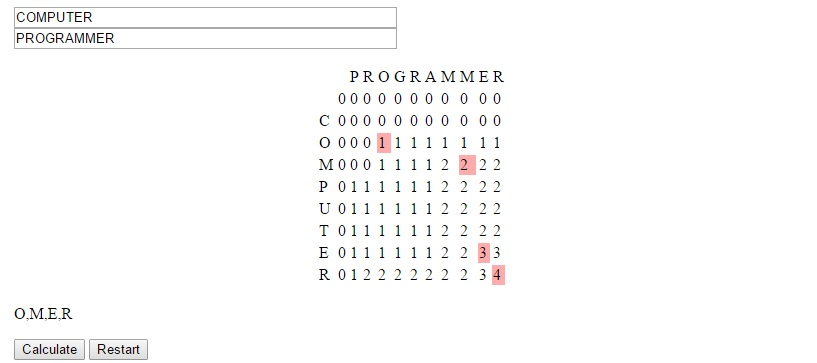The strategy used to devise this concept is called dynamic programming. It is useful we can solve larger problems by solving overlapping subproblems, as was the case here. In this situation we generally can store the data in a table form and avoid re-solving subproblems for which many larger problems will be dependent.

You can see this algorithm in action at LEARNINGlover.com: Longest Common Subsequece. Let me know what you think.

# Unidirectional TSP Puzzles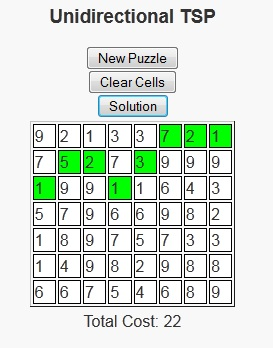As we’ve entered the late spring into early summer season, I’ve found myself wanting to go out more to sit and enjoy the weather. One of these days recently I sat in the park with a good book. On this occurrence, I decided not to go with a novel as I had just finished “Incarceron“, “The Archer’s Tale“, and “14 Stones” – all of which were good reads, but I felt like taking a break from the novels.

Just as a side note, 14 Stones is a free book available on smashwords.com and I’ve now read about 6 books from smashwords.com and haven’t been disappointed yet. My favorite is still probably “The Hero’s Chamber” because of the imagery of the book, but there are some well written ebooks available there by some good up and coming writers for a reasonable price, with some being free.

So with the desire to read, but not being in the mood for novels I decided to pick up one of my non-text but still educational books that make me think. This day it was “Programming Challenges“. I browsed through the book until I found one that I could lay back, look at the water, and think about how to solve it.

The programming puzzle the peaked my interest was called “Unidirectional TSP”. We are given a grid with m rows and n columns, with each cell showing the cost of using that cell. The user is allowed to begin in any cell in the first column and is asked to reach any cell in the last column using some minimum cost path. There is an additional constraint that once a cell is selected in a column, a cell in the next column can only be chosen from the row directly above, the same row, or the row directly below. There is a javascript version of this puzzle available here.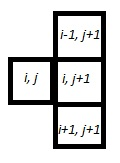Fundamentally, the problem is asking for a path of shortest length. Many shortest length problems have a greedy structure, but this one gained my interest because the greedy solution is not always optimal in this case. So I took a moment to figure out the strategy behind these problems. Once I had that solution, I decided that it would be a good program to write up as a puzzle.

In this puzzle version, users will click the cells they wish to travel in each column in which case they will turn green (clicking again will turn them white again). Once the user clicks on a cell in the last column, they will be notified of whether or not they have chosen the minimum path. Or if users are unable to solve a puzzle, the “Solution” button can be pressed to show the optimal path and its cost.

# Triangle Sum Puzzle

This is probably a consequence of being a mathematician, but I have always enjoyed number puzzles. I think that there is a general simplicity and universality in numbers that are not present in things like word puzzles, where the ability to reach a solution can be limited to the vocabulary of the user.

The fact that these are puzzles and not simply homework exercises also helps because we often find people sharing difficulties and successes stories over the water cooler or at the lunch table. The fact that many of these math puzzles can teach some of the same concepts as homework problems (in a more fun and inclusive way) is generally lost on the user as their primary interest is generally on solving the puzzle in front of them, or sometimes solving the more general form of the puzzle.

Today’s post is about a puzzle that was originally shared with me over a lunch table by a friend who thought it was an interesting problem and asked what I thought about it. I didn’t give the puzzle much further thought (he had correctly solved the puzzle) until I saw it again in “Algorithmic Puzzles” by Anany Levitin and Maria Levitin. It was then that I thought about the more general form of the puzzle, derived a solution for the problem, and decided to code it up as a script for my site.

Below is a link to the puzzle: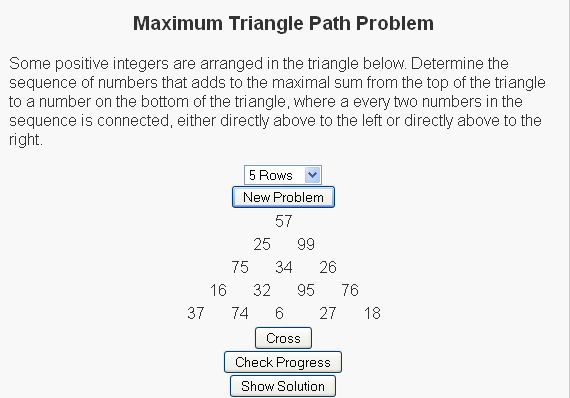We have a set of random numbers arranged in a triangle and the question is to find the path of maximum sum from the root node (the top node) to the base (one of the nodes on the bottom row) with the rules that
(1) Exactly one number must be selected from each row
(2) A number can only be selected from a row if (a) it is the root node or (b) one of the two nodes above it has been selected.

For the sample
So for the sample problem in the picture, the maximal path would go through nodes 57, 99, 34, 95, and 27.

For more of these puzzles check out the script I write here and be sure to let me know what you think.

# Assembly Line Scheduling

I wanted to take a minute to help some users become more familiar with Dynamic Programming, so I decided to write a script on the Assembly Line Scheduling Problem.

To introduce the problem I want to tell you a story about a friend of mine. Keisha recently started a clothing company that uses two assembly lines to produce articles of clothing. She has separated the the process of manufacturing an item of clothing into n steps, so each assembly line is separated into n different stations, with each station performing a specific task (So for example station three’s job may be to add a right sleeve to shirts). The task of a specific station is independent of which line the station occurs on (so if station three’s job is to add a right sleeve to shirts, this will be true in both assembly line 1 and assembly line 2). Lets denote the jth station (with j = 1, 2, …, n) on line i (where i is 1 or 2) by Si, j. Although they’re doing the same jobs the time it takes the employee at station S1, j may be different from the time it takes the employee at station S2, j. We will denote the time required at station Si, j by ai, j. For each line, there is also an amount of time required for the article of clothing to enter assembly line i, ei; and an amount of time required for the article of clothing to exit assembly line i, xi.

One of the reasons that assembly lines are very productive is that stations on the same assembly line are generally in close proximity to one another, resulting in a very low cost of transferring an item from one station to the next on the same assembly line. When we have multiple lines in place, as Keisha has, there is a (possibly beneficial) cost of transferring an item from one line to another. Lets denote this cost by ti, j which represents the cost of transferring a partially completed item of clothing from line i after having gone through station Si, j (again, i is 1 or 2 and j = 1, 2, …, n).

The problem that Keisha would like solved is to determine which station to choose between lines 1 and 2 in order to minimize the total time it takes to produce an article of clothing.

Consider the following example:Our goal is to get the clothing through the 3 states to produce a final product. What if we initially had the product take the route through station S2, 1 instead of station S1, 1? Lets assume that we make the decisions to send the article of clothing to stations S2, 2 and S2, 3 afterwards. This would result in a solution whose total cost is 3 + 8 + 4 + 6 + 3 = 24. Is this solution optimal (aka is this solution the minimum total time through the factory)? Lets consider what would happen if we had chosen station S1, 1 instead of S2, 1. The entry cost for line 1 is 1, the time required at station S1, 1 is 5 and the transfer time to go to assembly line 2 is 1. So the cost of this new solution is 1 + 5 + 1 + 4 + 6 + 3 = 20, which gives a cheaper solution.

This is called the principle of optimality (optimal substructure property) which states that in order for an overall solution to be optimal, the solution must also give the optimal solutions to every subproblem of the original problem. This problem of solving all subproblems may seem like a daunting task at first, but lets consider the example above again.

Initially, we have a new product and there are two options – either line one or line two. We will need these values in the future, so lets keep track of both choices in the form of a table.

 Station 1 cost0 e1 + a1, 1 cost1 e2 + a2, 1

After this initial step, the question becomes given the current path to station j-1, which assembly line can best serve station j? This cam be computed for each j > 1 by
cost1(j) = min{cost1(j-1) + a1, j, cost2(j-1) + t2, j-1 + a1, j}
cost2(j) = min{cost2(j-1) + a2, j, cost1(j-1) + t1, j-1 + a2, j}

As you can see, the calculation of costi(j) relies on the computation of costi(j-1). By calculating these values from station 1 to to station n, we are able to simply look up the values in the table instead of having to recalculate these values.

These give optimal solutions to each of the subproblems. We repeat this same step for all stages j = 2, …, n then we arrive at the final step were we finish the job. Lets define total_cost to indicate the cost of the optimal solution.
total_cost = min{cost1(n) + x1, cost2(n) + x2}

We’d like to see which value minimizes total_cost. Then we can trace back to find the values that minimized cost1 or cost2 at each step depending on which assembly line was chosen. The following algorithm does just this, and stores the assembly line chosen at each state in the variable line.

For the above example, the table would be calculated as follows:

 Station 1 Station 2 Station 3 Total Cost cost1 6 13 18 21 cost2 11 11 17 20

We can reconstruct the optimal path through assembly lines by seeing that we finish by going through station S2, 3.
We arrive at station S2, 3 by going through the assembly line station S2, 2.
We arrive at station S2, 2 by going through the assembly line station S1, 1.

This is precisely the path that is highlighted in the image above.

The algorithm to construct these paths and compute the total_cost for such problems is given below.

Algorithm FastestWay(a, t, e, x, m)
cost1 [<-] e1 + a1, 1
cost2 [<-] e2 + a2, 1
for (j [<-] 2 to n) if (cost1(j-1) + a1, j [<=] cost2(j-1) + t2, j-1 + a1, j
cost1(j) [<-] cost1(j-1) + a1, j
line1(j) [<-] 1 else cost1(j) [<-] cost2(j-1) + t2, j-1 + a1, j
line1(j) [<-] 2if (cost2(j-1) + a2, j [<=] cost1(j-1) + t1, j-1 + a2, j
cost2(j) [<-] cost2(j-1) + a2, j
line2(j) [<-] 1 else cost2(j) [<-] cost1(j-1) + t1, j-1 + a2, j
line2(j) [<-] 2if (cost1(n) + x1 [<=] cost2(n) + x2)
total_cost = cost1(n) + x1
final_line = 1
else
total_cost = cost2(n) + x2
final_line = 2